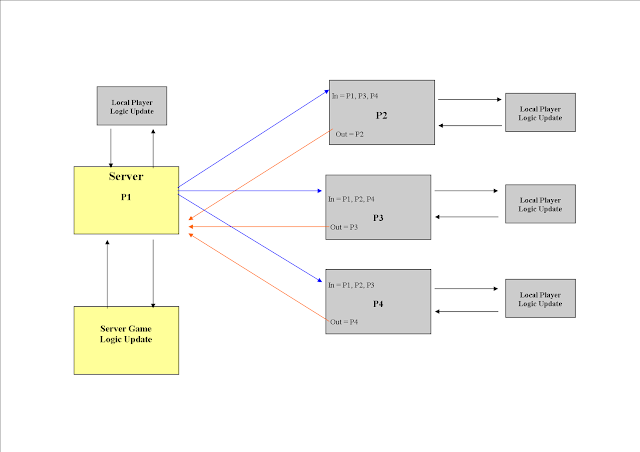## Thursday, 10 October 2013

### Finding the relative position of a point to a players orientation...

So for many things in a 3D game we need to convert the Relative Offset position of one object to another based on how one of the objects would see the other one!!!

Example.

Player 1 and Player 2 are near to each other.  Player 1 is at 0,0 and Player 2 is at 100,5.
Player 1 is facing towards 0 degrees or North.  So from Player 1`s point of view then Player 2 is to his right by 100 and in front by 5 units.
But IF Player 1 turns 90 degrees to his left then Player 2s new relative position to Player 1 becomes 5,-100.

You can see that the X and Y relative coordinates have changed based on which direction Player 1 is facing.

The best way to think of this is light a Radar on a vehicle (in our case a Submarine).  The dots on the radar are Always Relative to the Orientation of the vehicle.

Why?

Well it turns out that (apart from having a Radar) it is a lot easier to check for collision between 2 objects by using simple Point within a Box Area check, than for checking if a single point is Anywhere Within a Box Area that could be pointing in any 3 Dimensional direction!

We can rotate the Point to relative to the Player, which basically means the Player is Always pointing at 0 degrees ie North. And only the Relative point moves around the player.

Here is the actual code function from our game that does this:-

Vector3 Find_Relative_Position(Matrix R_Orientation, Vector3 R_Position)
{

Vector3 Xaxis = R_Orientation.Right;

Vector3 Yaxis = R_Orientation.Up;

Vector3 Zaxis = R_Orientation.Forward;

float Rx = (Xaxis.X * R_Position.X) + (Xaxis.Y * R_Position.Y) + (Xaxis.Z * R_Position.Z);

float Ry = (Yaxis.X * R_Position.X) + (Yaxis.Y * R_Position.Y) + (Yaxis.Z * R_Position.Z);

float Rz = (Zaxis.X * R_Position.X) + (Zaxis.Y * R_Position.Y) + (Zaxis.Z * R_Position.Z);

return new Vector3(Rx, Ry, -Rz);

}

Just send it the Orientation Matrix of the Viewer (ie Player that you want to check collision with) and also the Point relative to the viewers position (ie Player Position - Point Position)

The above function will then return the Relative Point vector in respect to the Players Orientation View.

## Thursday, 3 October 2013

### Concept Videos

Still early build...

Very early testing...

## Network diagram for Deep Waters game on Xbox 360 Indie games.As you can see from the above diagram.  We have a Server-Client based network setup.

After trial and error and a few calculations we have decided that the above is the most efficient way for this particular game.

Key points:-

Each player controls his own submarine.

Each player sends their submarine data to the Server.

The Server sends it`s own and the other remaining players in the network data to each of the other players.

The Server Can choose to override Any players data if needs be, ie at Initialization and players death.

Generally the server does not update the local players data. ie Each client controls his own submarine directly.

This produces smooth controls for each of the local players.  We will then use predictive movements for all players, as viewed from each client player.

The formula for calculating the bandwidth is the following:-

Network bandwidth  =  (  (n-1)*(n-1) + (n-1)  )  *  DataPerPlayer (bytes)

( (n-1)*(n-1)+(n-1) ) happens to break down to (n*(n-1)), so for example 4 players would need 4*3 = 12 lots of network data to sync all 4 players in the network.

In our example of 4 players (n) and each player needing 20 bytes of data to sync their position and orientation means we need 4x3 = 12 x 20 = 240 bytes per frame. If we update our game network at 20 frames per second (fps) then in 1 seconds our bandwidth usage will be 4x3x20x20 = 4800 bytes per second (bps). If we forget about the headers that is!!!